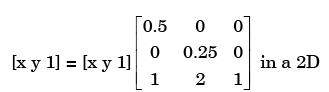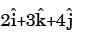Courses

# Past Year Questions: CIMS (CAD & CAM) Notes | EduRev

## Mechanical Engineering : Past Year Questions: CIMS (CAD & CAM) Notes | EduRev

The document Past Year Questions: CIMS (CAD & CAM) Notes | EduRev is a part of the Mechanical Engineering Course Manufacturing and Industrial Engineering.
All you need of Mechanical Engineering at this link: Mechanical Engineering

Question 1:In a CAD package, mirror image of a 2D point P(5, 10) is to be obtained about a line which passes through the origin and makes an angle of 45° counterclockwise with the X-axis coordinates of the transformed point will be



Question 2:The geometric transformation specified byIn a 2D CAD system representsQuestion 3:In a CAD package, a point P(6,3,2) is projected along a vector V r (-2,1,-1). The projection ofthis point on X-Y plane will be



Question 4:Complete the manual part program for drilling a hole located at point (20, 20) from the target point. The depth of the hole to be drilled is 15 mm FROM/TARG
GOTO/20, 20, 0



Question 5:Given that the notations of robot joints are Llinear, O-orthogonal, R-rotation, T-twisting, and V- revolving, the anatomy of a polar configuration robot is described by the alphabetical string



Question 6:The component development and manufacturing activities when assisted by a computer area: computer aided graphics (CAG), CAD, CAM, CAPP and computer aided quality control (CAQC).The most appropriate sequence of these activities is



Question 7:The transformation matrix for mirroring a point in x-y plane about the line y = x is given by

Question 8:A point P(1, 3, -5) is translated byand then rotated counter clockwise by 90° about the z-axis. The new position of the point is:



Question 9:For the situation shown in the figure below the expression for H in terms of r, R and D is

Question 10:The figure below represents a triangle PQR with initial coordinates of the vertices as P(1, 3), Q(4, 5) and ft(5.3.5). The triangle is rotated in X-Y plane about the vertex P by angle q in clockwise direction. If sinq = 0.6 and cosq = 0.8, the new coordinates of the vertex Q are



Question 11: A triangular face in a CAD model has vertices: P1(0, 0, 0); P2(1, 1, 0) and P3(1,1, 1).The area of the facet is



Question 12:A robot arm PQ with end coordinates P(0,0) and Q(2,5) rotates counter clockwise about P in the XY plane by 90°. The new coordinate pair of the end point Q is



Question 13:For generating a Coon's surface we require



Question 14: In a 2–D CAD package, clockwise circular arc of radius 5, specified from P1 (15, 10) to P2 (10, 15) will have its center at



Question 15:In computer aided drafting practice, an arc is defined by



Offer running on EduRev: Apply code STAYHOME200 to get INR 200 off on our premium plan EduRev Infinity!

## Manufacturing and Industrial Engineering

91 videos|52 docs|59 tests

,

,

,

,

,

,

,

,

,

,

,

,

,

,

,

,

,

,

,

,

,

;